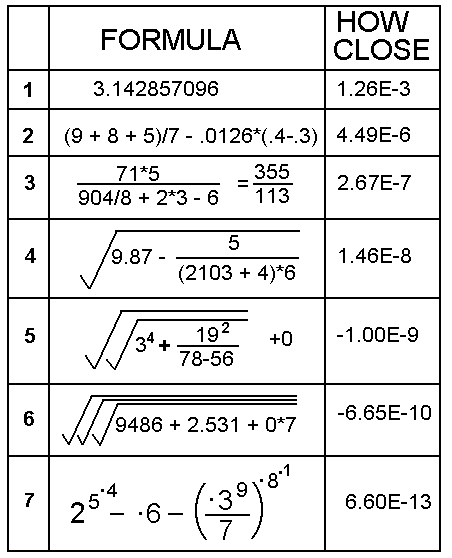## PI IN 10 DIGITS

The puzzle this month is to find a numerical expression that is as close as possible to pi using the numerals 0 to 9 once and once only. You may use any number of the four arithmetic operators (+ - × ÷) plus decimal point, brackets and the square root sign but no other symbols or letters. Oblique stroke can be used for divide. Exponentiation is allowed.

Example:      (9 + 8 + 5) / 7 + 0 x (1.2346) = 22 / 7

22 / 7 is too big by 0.00126. Can you do better?

The competitor with the closest approximation is the winner.SOME ANSWERS

I set this question not knowing that it was not original, as I found out from the website mathworld.wolfram/PiApproximations.html where the problem is referred to as a "pandigit" approximation.

Some solutions in order of increasing accuracy are shown in the graphic opposite (using graphics enables me to use mathematical symbols and proper mathematical layout).

Number 1 is from Colin Row who made the mistake of assuming that pi was 22/7. If it were, my example formula above would be 100% accurate.

Number 2 is a good effort from Michael Hodge whose error was as little as 4.49E-6.

I thought that someone would try to get the next closest rational approximation after 22/7 which is 355/113. Number 3 is one example of many using all ten digits.

Number 4 is from Richard Burkill who found an approximation as close as 1.46E-8. Well done.

Number 5 was found on the internet (at the url given above) which gets the approximation down to -1E-9 which is pretty good.

This problem was one of the rare occasions where the problem setter is on equal terms with the solvers so I had a go and got number 6 which is 6.65E-10 too small. The hanging '7' and zero was an embarrassment -- it would have been nice for these to be included in the main formula but I was unable to find a way. Multiplying them together and adding was an ugly way to make the answer stick to the rules.

Number 7 is incredibly accurate: one thousand times more accurate than my own number 6. This was found on the Internet and also submitted by John Stafford and acknowledged to be atributed to Richard Hess. I had to include it for the challenge of drawing the formula! (I have assumed that where there are multiple exponentiation operators the precedence is from right to left, which is usual.)

So in conclusion I award the prize this month to Richard Burkill as the best of the club solvers with special mention to John Stafford who sent me a large number of interesting solutions.

[home] [back]